HomeBlogSoftware DevelopmentHow to Add Two Numbers in Java?

# How to Add Two Numbers in Java?

Last updated:
4th Jun, 2023
Views
5 MinsView AllGiven below is the flowchart depicting the addition of two numbers. The process is very simple. We are supposed to input two numbers and add them up. Save the answer in Sum and display the result.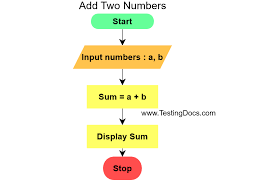Now we will take the next jump and see two programs for the addition of two numbers in Java. In the first example, we assign a value of the two numbers within the code. The second program takes the two numbers passed by the user and displays the sum.

## How To Add Two Numbers In Java?

In Java, adding two numbers requires adding two integers and printing the result. Adding two numbers in the Java programming language is straightforward; let’s look at the algorithm.

### Algorithm

Below mentioned is the most basic algorithm for the addition of two numbers in Java :

• Introduce two variables.
• Set some values as initial values for the variables.
• Set up a second variable to hold the total of the numbers.
• Add two values together using the plus (+) operator in mathematics, then save the outcome.

### Sum Of Two Numbers Using Method

Here are two methods to add two numbers in Java:

• Using sum() method
• Using User-defined method

### sum() method

sum() is a function available in the integer class. Given below is a simple Java program to add two numbers using the sum() method.

Example code:

{

public static void main(String args[])

{

int m = 1, m = 2, x = 3, y = 4, reqsum1, req_sum2;

reqsum1=Integer.sum(m, n);

System.out.println(“Given sum of m and n is: ” +req_sum1);

reqsum2=Integer.sum(x, y);

System.out.println(“Given sum of x and y is: ” +req_sum2);

}

}

Output:

Given sum of m and n is: 3

Given sum of x and y is: 7

### User-defined method

The user can read input in Java and enter two numbers. Next, they will send it to the user-defined method sum().

The Java program to add two numbers using a user-defined method is implemented below.

{

public static void main(String args[])

{

int a, b, req_sum;

Scanner sc = new Scanner(System.in);

a = sc.nextInt();

b = sc.nextInt();

req_sum = final_sum(a, b);

System.out.println(“Sum of numbers ” + a + ” and ” + b + ” is: ” +reqsum );

}

public static int final_sum(int m, int n)

{

int final_sum = m + n;

return final_sum;

}

}

### Sum Of 3 Numbers

The sole difference between the sum of three numbers and the sum of two numbers is that there are three variables in this case.

### Sum Of N Numbers

This method of figuring out the sum of ‘N’ numbers is more inclusive. Below is the general algorithm to find the sum of ‘N’ numbers in Java.

• The number of integers to be added, N, should be initialised.
• The loop will repeat N times and prompt the user for input, or it may not, depending on how the loop is initialised.
• Put the sum of each input’s values into a variable.
• Once all iterations have been completed, print the sum value.

Check out our free technology courses to get an edge over the competition.

## Example 1: Finding the sum of two numbers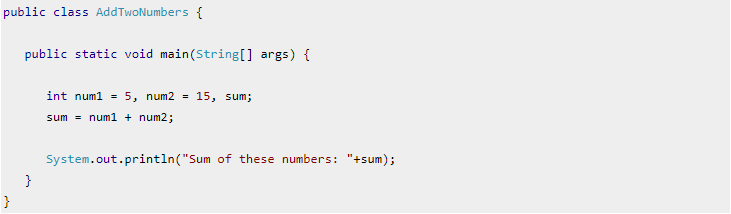Output: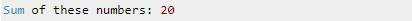If we observe in the above-given program, we’ll find out that the values of the numbers are specified in the program.

Check Out upGrad’s Software Development Courses

## Example 2: Use of Scanner to compute the sum of two numbers

### What is a scanner?

The scanner helps us to capture the input given by the user so that the values of the two numbers can be obtained from the user. Then, the program computes the sum and shows it as output.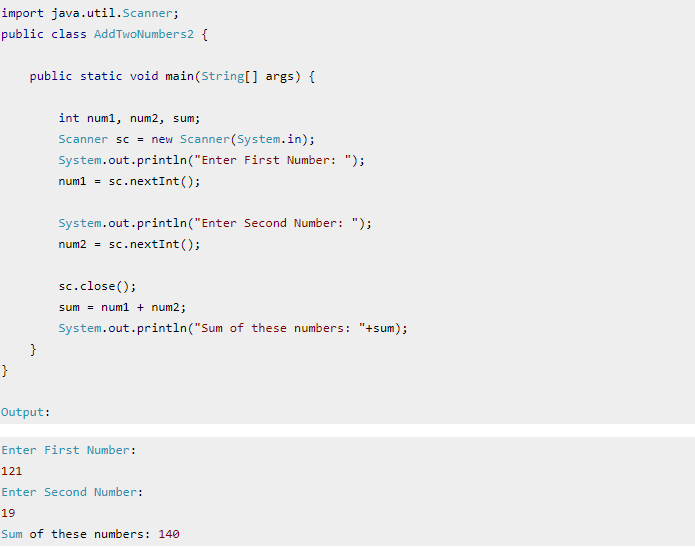## Explore our Popular Software Engineering Courses

 Master of Science in Computer Science from LJMU & IIITB Caltech CTME Cybersecurity Certificate Program Full Stack Development Bootcamp PG Program in Blockchain Executive PG Program in Full Stack Development View All our Courses Below Software Engineering Courses

If we see in the above program, we’ll find that at the end of the program, both the numbers are required to be furnished by the user and then the sum is calculated and displayed at the end.

## Third Example: Sum of two numbers Using command line arguments

The aforementioned method is not the only way to add two numbers. You can add two number using command line arguments as well. Check out the program given below to learn and understand how to add two numbers with command line arguments: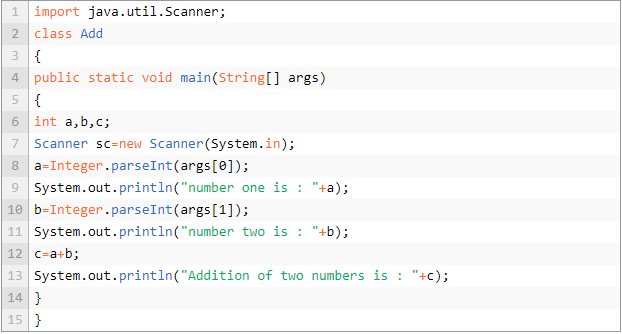## In-Demand Software Development Skills

 JavaScript Courses Core Java Courses Data Structures Courses Node.js Courses SQL Courses Full stack development Courses NFT Courses DevOps Courses Big Data Courses React.js Courses Cyber Security Courses Cloud Computing Courses Database Design Courses Python Courses Cryptocurrency Courses

Output :If you want to learn how to add two numbers in Java, you can use the aforementioned methods. There are other methods as well that you can use to do the same, like Using Buffered Reader, Using Method, Without Using Addition+ Operator, & Using Classes and Objects.

At upGrad, we understand the importance of practical, hands-on learning – especially when it comes to software development. As a result, our courses and training initiatives have practicality at their very core. One such initiative is Full Stack Development Bootcamp which will help you develop all the relevant skills required to excel in full-stack development.Blog Author
Director of Engineering @ upGrad. Motivated to leverage technology to solve problems. Seasoned leader for startups and fast moving orgs. Working on solving problems of scale and long term technology strategy.
Get Free Consultation

Select CourseSelectBy clicking 'Submit' you Agree to